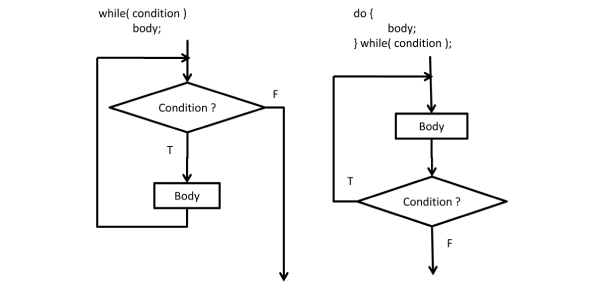# C Loops: For, While, Do While Quiz! Test

14 Questions | Attempts: 5615SettingsC is an amazing language that is easy to grasp at the same time. Take this C Loops: For, While, Do While Quiz! Test yourself today with the help of these carefully designed questions and find out just how much you know about creating loops in C. Don't forget to share this quiz with your programmer friends so they can try and answer these questions and see how well they did too! All the best!

• 1.
What is the output? for(int i=1; i<15; i=i+3)   {         out.print(i); }
• A.

15

• B.

1471013

• C.

47101316

• D.

147101516

• 2.
What represents the condition?for(int j=11; j>-2; j=j-2)
• 3.
What is the value of m when you exit the loop?for(int m=30; m>0; m=m-4)   {         out.println(m); }
• 4.
What is the output?int total=0; for(int s=1; s<15; s++)   {         total=total+s; } out.println(total);
• 5.
What is the output?for(int m=30; m>0; m=m-4)   {         out.print(m); }
• 6.
What is the output"for(int x=20; x<40; x=x+3)   {         out.print(x); }
• 7.
What is the start point of the loop?(just the number)for(int x=20; x<40; x=x+3)   {         out.println(x); }
• 8.
What is the output?int x=1; while(x<5) {    x++;    System.out.print(x); }
• 9.
What is the output?int m=2, total=0; while(m<6) {    total=total+m;    m++; } System.out.print(total);
• 10.
What is the output?int z=2, sum=0; while(z<9) {    z++;    sum=sum+z; } System.out.print(sum);
• 11.
What is the output?int k=3; String s=""; while(k>-1){   s=k+" "+s;   k--; } System.out.print(s);
• 12.
What is the output?int b=5; String list=""; while(b<11) {    b=b+2;    if(b%2==1)      list=b+" "+list; } System.out.print(list);
• 13.
What is the output?int x=1; do{    x++;    System.out.print(x); }while(x<5);
• 14.
What is the output?int k=3, tot=0; do{    tot=tot+k;    k++; }while(k<11); System.out.print(tot);

## Related TopicsBack to top
×

Wait!
Here's an interesting quiz for you.